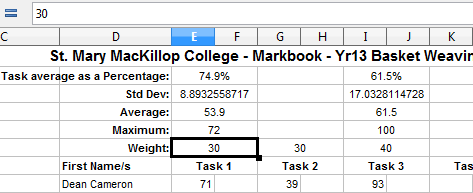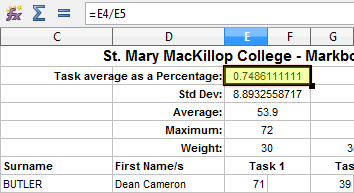Okay, all that data at the top of the sheet needs completing so let’s attack it piece by piece:Std Dev?!?
So what is Std Dev you may be asking, it simply stands for Standard Deviation and if the whole concept of Standard Deviation (or SD) is new to you then without getting into the complicated maths, it basically gives you the “deviation” to the average that is considered “standard” for the range of results in that average. In order to work it out for a range you simply use the STDEVP function, i.e:

`STDEVP(E8:E37)`

This number is important when determining Z-Scores which we will be addressing later. For now though, see if you can get this formula to be executed on the condition there is at least two task results to work with and that there is a maximum score included.